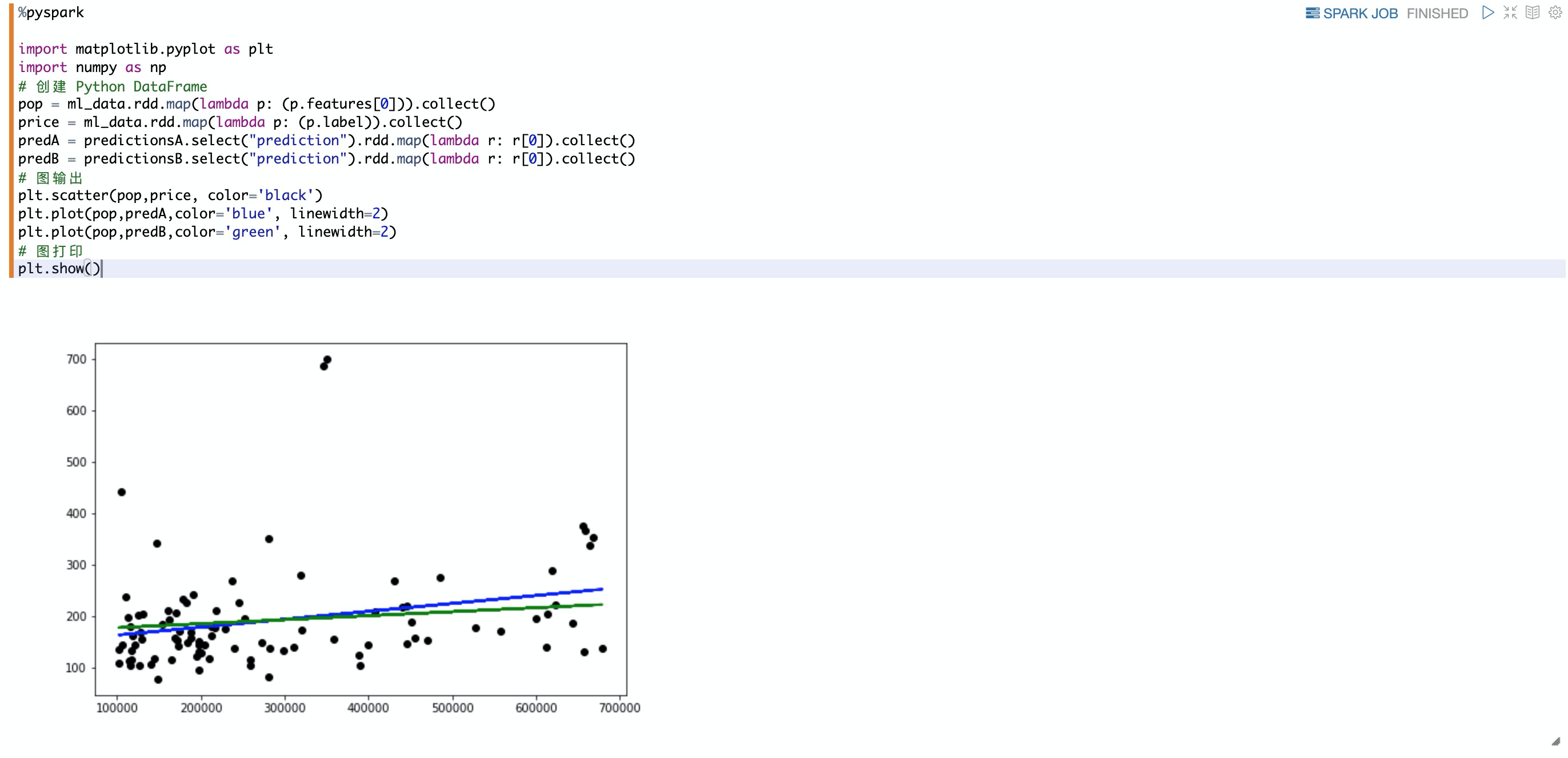# Databricks数据洞察机器学习

## 前提条件

DDI访问OSS路径结构：oss://BucketName/Object

• BucketName为您的存储空间名称。

• Object为上传到OSS上的文件的访问路径。

``````// 从oss地址读取文本文档
val dataRDD = sc.textFile("oss://databricks-demo-hangzhou/demo/The_Sorrows_of_Young_Werther.txt")``````

## 开发流程

1. 创建集群并通过knox账号访问Notebook

2. 创建Notebook、加载样本数据

3. 准备ML算法数据

4. 建立模型、运行线性回归模型

5. 评估线性回归模型

6. 可视化线性回归模型

## 步骤二：创建Notebook、加载样本数据

1.加载oss数据，并缓存

``````%pyspark
path="oss://databricks-datasets/samples/data_geo.csv"  # 根据oss路径；
# 加载样本数据并打印；
.option("inferSchema", "true") \
.csv(path) \

data.cache() # 缓存数据以加快重用``````

2.数据展示，打印schema

``````%pyspark
data.show(5)
data.printSchema()``````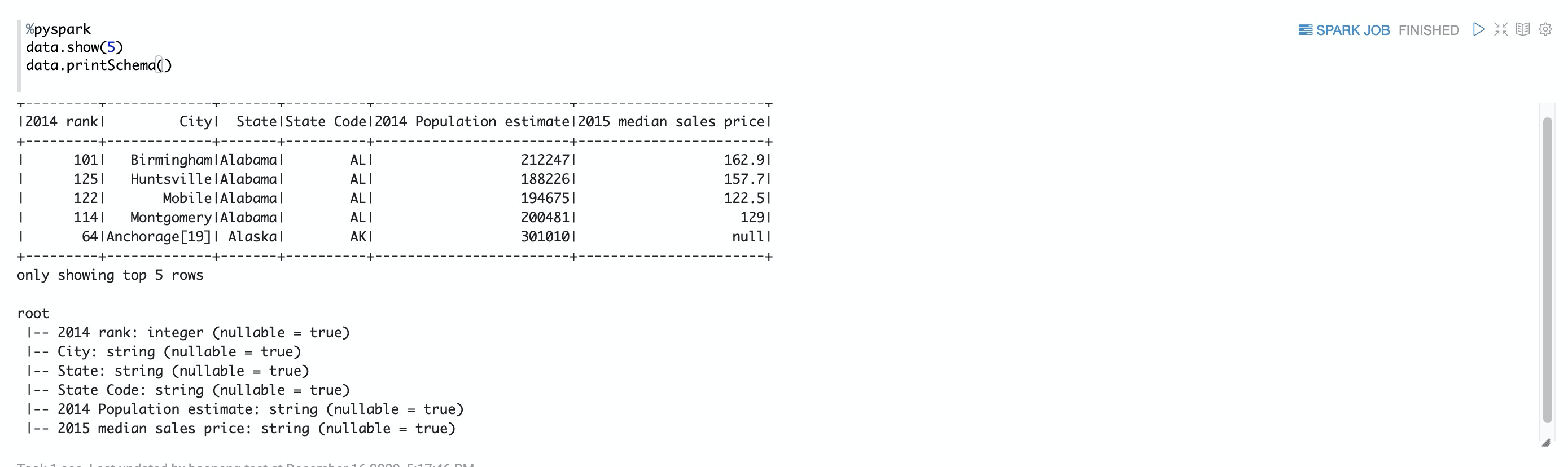## 步骤三：准备ML算法数据

1.数据类型转换，删除缺少值的行，然后重命名特征和标签列，并用"_"替换空格

``````%pyspark

from pyspark.sql.functions import col
from pyspark.sql.types import DoubleType
#数据类型转换
datat=data.select(col("2014 rank"),col("city"),col("state"),col("State Code"),col("2014 Population estimate"),col("2015 median sales price").cast(DoubleType()))
#删除缺少值的行
datat = datat.dropna()
#重命名特征和标签列，并用"_"替换空格
exprs = [col(column).alias(column.replace(' ', '_')) for column in datat.columns]``````

2.为了简化特征的创建，请注册UDF以将特征（2014_Population_estimate）列向量转换为VectorUDT类型并将其应用于该列

``````%pyspark

from pyspark.ml.linalg import Vectors, VectorUDT
# 定义UDF函数，数据向量化处理；
spark.udf.register("oneElementVec", lambda d: Vectors.dense([d]), returnType=VectorUDT())
ml_data = datat.select(*exprs).selectExpr("oneElementVec(2014_Population_estimate) as features", "2015_median_sales_price as label")
``````

3.数据展示

``````%pyspark
# 数据展示
ml_data.show()``````

ML算法数据打印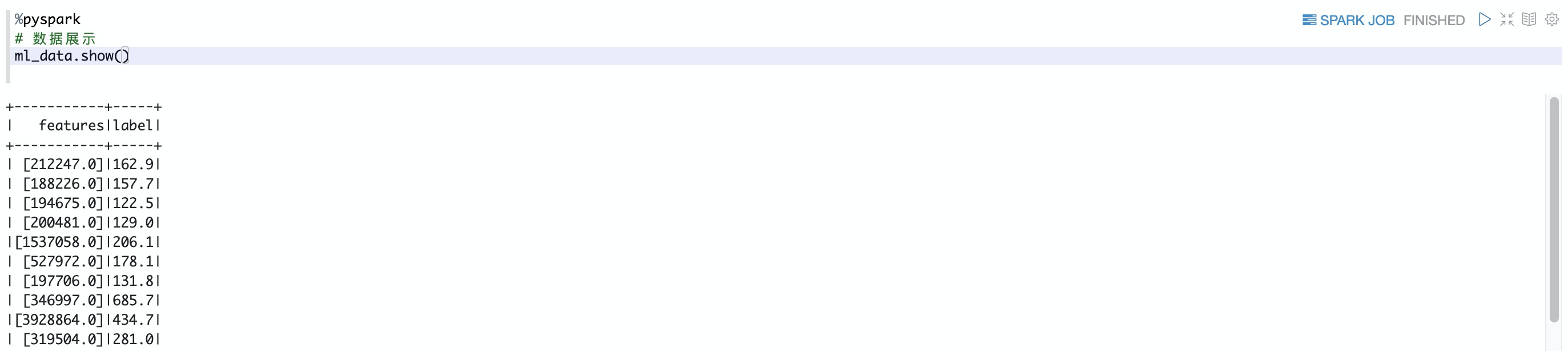## 步骤四：建立模型、运行线性回归模

1.建立模型

``````%pyspark

from pyspark.ml.regression import LinearRegression
# 定义LinearRegression算法
lr = LinearRegression()
# 建立模型
modelA = lr.fit(ml_data, {lr.regParam:0.0})
modelB = lr.fit(ml_data, {lr.regParam:100.0})
``````

2.使用该模型并作出预测，您可以使用transform()函数进行预测，该函数会添加新的预测列。例如下面的代码采用第一个模型（modelA），并根据特征（features）向您显示标签（原始销售价格）和预测（预测销售价格）

``````%pyspark

# 运行线性回归模型，并展示数据
predictionsA = modelA.transform(ml_data)
predictionsA.show(10)``````

## 步骤五：评估线性回归模型

``````%pyspark

from pyspark.ml.evaluation import RegressionEvaluator
# 使RegressionEvaluator用来计算均方根误差 。
evaluator = RegressionEvaluator(metricName="rmse")
RMSE = evaluator.evaluate(predictionsA)
print("ModelA: Root Mean Squared Error = " + str(RMSE))
# ModelA: Root Mean Squared Error = 128.602026843

predictionsB = modelB.transform(ml_data)
RMSE = evaluator.evaluate(predictionsB)
print("ModelB: Root Mean Squared Error = " + str(RMSE))
# ModelB: Root Mean Squared Error = 129.496300193``````

RMSE评估结果打印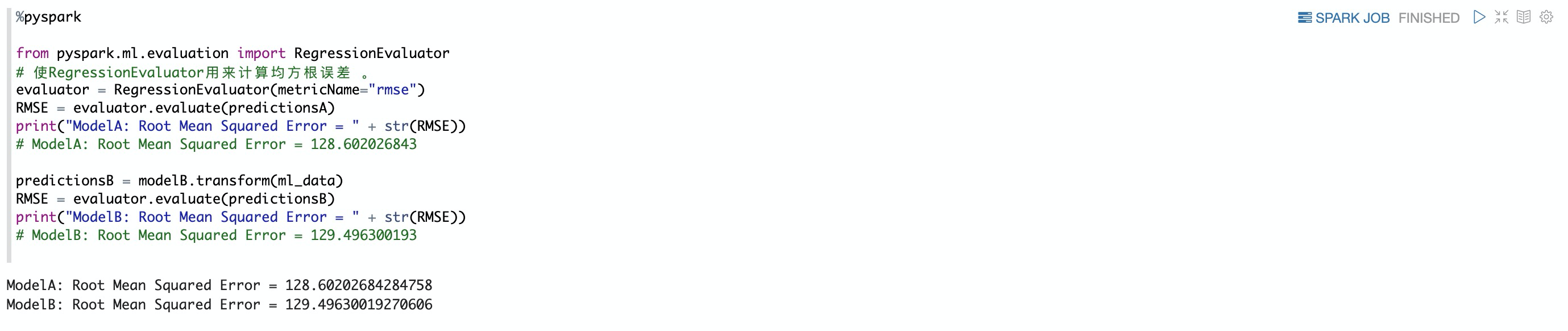## 步骤六：可视化线性回归模型

1. 依赖库导入

1. 当使用pyspark进行开发时候，依赖的库可以通过ddi库能力导入，具体见：https://help.aliyun.com/document_detail/168087.html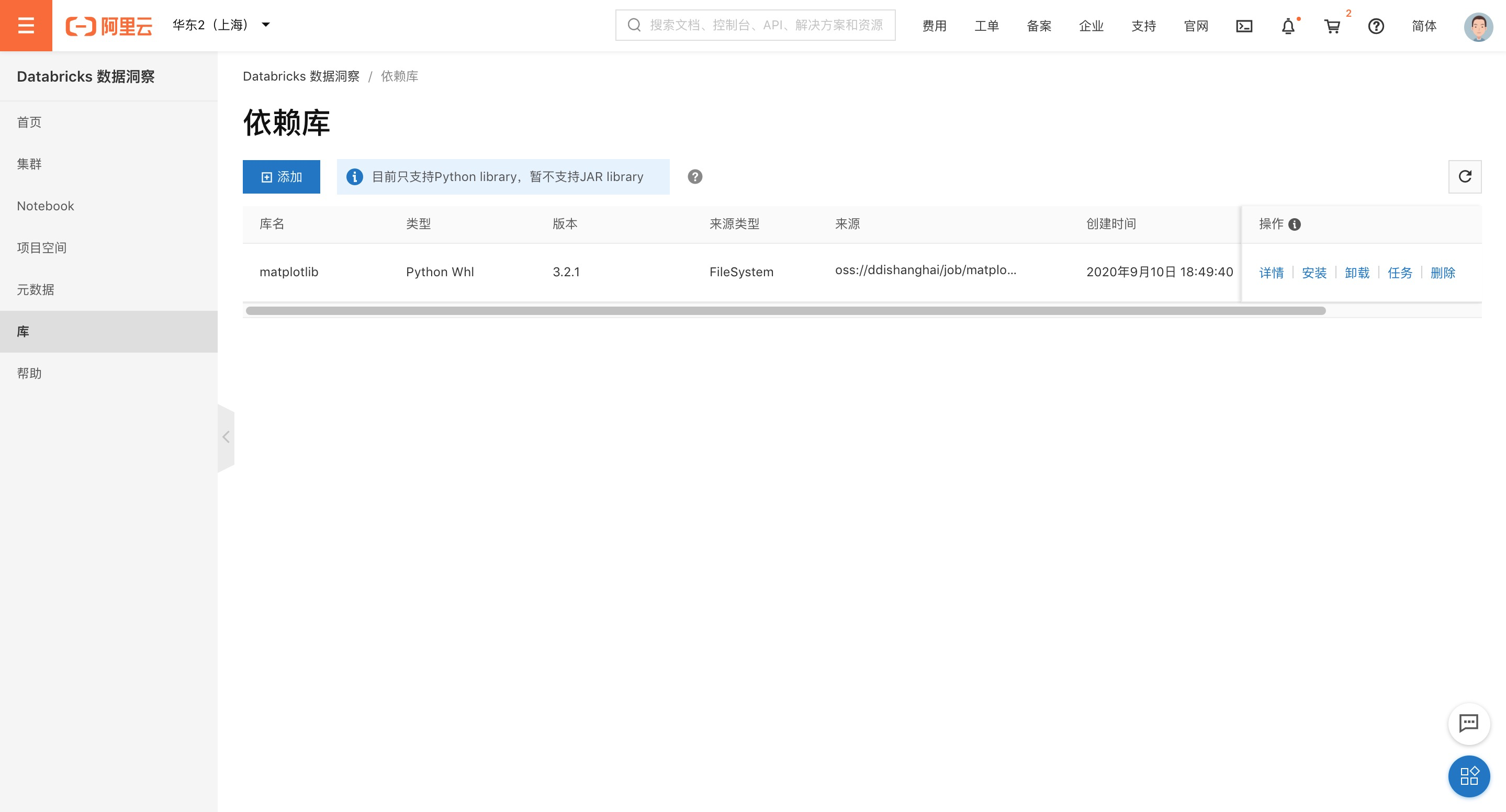2.创建Python DataFrame 、使用matplotlib做图渲染 、图打印

``````%pyspark
#为展示效果，本实例对样本数据做了部分筛选；
import matplotlib.pyplot as plt
import numpy as np
# 创建 Python DataFrame
pop = ml_data.rdd.map(lambda p: (p.features)).collect()
price = ml_data.rdd.map(lambda p: (p.label)).collect()
predA = predictionsA.select("prediction").rdd.map(lambda r: r).collect()
predB = predictionsB.select("prediction").rdd.map(lambda r: r).collect()
# 图渲染
plt.scatter(pop,price, color='black')
plt.plot(pop,predA,color='blue', linewidth=2)
plt.plot(pop,predB,color='green', linewidth=2)
# 图打印
z.show(plt)``````